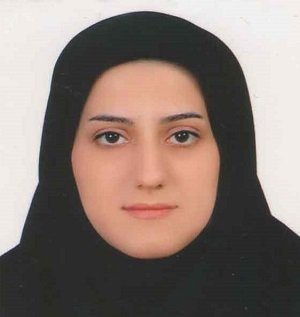دوشنبه, 15 آذر,1400 فارسی
•• [فارسی]

# Haleh Tajadodi ، Assistant Professor

Faculty : Math
Departments : Mathematics and its applications
Phone : +98 054 - 31136371
• 2018 2010

MS , Applied Mathematics - Numerical Analysis , University of Mazandaran , 2008 → 2010
PhD , Applied Mathematics - Numerical Analysis , University of Mazandaran , 2011 → 2014

## Solving fractional differential equations based on operational matrices

محمود شيبك , [ Haleh Tajadodi ]
نيمسال اول سال تحصيلي 97-96

1 - Hossein Jafari, Haleh Tajadodi, New method for solving a class of fractional partial differential equations with applications, Thermal Science. (2018) 22 277-286
2 - - -, , Haleh Tajadodi, , Analysis of Riccati Differential Equations within a New Fractional Derivative without Singular Kernel, FUNDAMENTA INFORMATICAE. (2017) 151 161-171
3 - - -, Haleh Tajadodi, Electro-spun organic nanofibers elaboration process investigations using BPs Operational Matrices, iranian journal of mathematical chemistry. (2016) 7 19-27
4 - - -, Haleh Tajadodi, Sarah Jane JOHNSTON, A decomposition method for solving diffusion equations via local fractional time derivative, Thermal Science. (2015) 19 123-129
5 - Hossein Jafari, Haleh Tajadodi, - -, A numerical approach for fractional order Riccati differential equation using B-spline operational matrix, Fractional Calculus and Applied Analysis. (2015) 18 387-399
6 - Hosain Jafari, Haleh Tajadodi, , Application of a homogeneous balance method to exact solutions of nonlinear fractional evolution equations, Journal of Computational and Nonlinear Dynamics. (2014) 9 021019-1-021019-4
7 - - -, Haleh Tajadodi, FRACTIONAL ORDER OPTIMAL CONTROL PROBLEMS VIA THE OPERATIONAL MATRICES OF BERNSTEIN POLYNOMIALS, University Politehnica of Bucharest Scientific Bulletin-Series A-Applied Mathematics and Physics. (2014) 76 115-128
8 - - -, Haleh Tajadodi, - -, , , , EXACT SOLUTIONS OF BOUSSINESQ AND KdV-mKdV EQUATIONS BY FRACTIONAL SUB-EQUATION METHOD, Romanian Reports in Physics. (2013) 65 1119-1124
9 - - -, Haleh Tajadodi, , - -, Fractional Subequation Method for Cahn-Hilliard and Klein-Gordon Equations, ABSTRACT AND APPLIED ANALYSIS. (2013) 2013 1-5
10 - - --, Haleh Tajadodi, - -, A modified variational iteration method for solving fractional Riccati differential equation by Adomian polynomials, Fractional Calculus and Applied Analysis. (2013) 16 109-122
11 - - -, , Haleh Tajadodi, , Homotopy analysis method for solving Abel differential equation of fractional order, CENTRAL EUROPEAN JOURNAL OF PHYSICS. (2013) 10 1523-1527
12 - - -, Haleh Tajadodi, , , , , Fractional sub-equation method for the fractional generalized reaction Duffing model and nonlinear fractional Sharma-Tasso-Olver equation, CENTRAL EUROPEAN JOURNAL OF PHYSICS. (2013) 11 1482-1486
13 - - -, , , Haleh Tajadodi, Numerical solution of fractional differential equations by using fractional B-spline, CENTRAL EUROPEAN JOURNAL OF PHYSICS. (2013) 11 1372-1376
14 - - -, Haleh Tajadodi, , Homotopy analysis method for solving a couple of evolution equations and comparison with Adomians decomposition method, Waves in Random and Complex Media. (2011) 21 657-667
15 - - -, , Haleh Tajadodi, Solving a multi-order fractional differential equation using homotopy analysis method, JOURNAL OF KING SAUD UNIVERSITY SCIENCE. (2011) 23 151-155
16 - - -, - -, Haleh Tajadodi, Convergence of Homotopy Perturbation Method for Solving Integral Equations, Thai Journal of Mathematics. (2010) 8 511-520